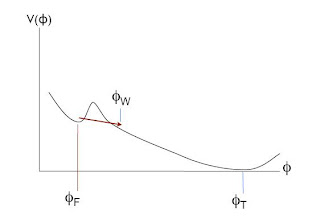## Thursday, December 02, 2010

### Is space both finite and infinite?This is an interesting diagram, for at least a couple of reasons. Firstly, as the author, cosmologist Anthony Aguirre explains in his paper Eternal Inflation, past and future, "it may well represent the current best bet for how the observable universe actually originated." Secondly, it demonstrates nicely how, according to general relativistic cosmology, the observable universe could be both spatially infinite and spatially finite.

Aguirre's diagram represents the creation of our observable universe according to a certain scenario proposed by inflationary cosmology, so let's begin by recapping the basic idea of the latter. Inflation suggests that there is a scalar field, the 'inflaton', whose 'equation of state' is such that a positive energy density corresponds to a negative pressure. In general relativity, a matter field with negative pressure generates a repulsive gravitational effect. Inflationary cosmology suggests that at some time in the early universe, the energy density of the universe came to be dominated by the non-zero energy density of the inflaton field. A region of the universe in this so-called false vacuum state would undergo exponential expansion until the inflaton field dropped into a lower energy state. This lower energy state is conventionally considered to be the 'true vacuum' state, the lowest energy state of the inflaton field.

The diagram above represents a particular type of inflationary scenario in which inflation ends locally at a 'nucleation point', by quantum tunnelling from the false vacuum value φF, to a value φW. An expanding bubble of lower-energy forms, the bubble wall expanding outwards at the speed of light. The bubble wall is duly represented on Aguirre's diagram by the vee-shape. (If one were to add an extra spatial dimension to the diagram, then one would represent the expanding bubble wall as a cone-shape).Whilst the bubble wall possesses an inflaton field value of φW, the region inside the bubble evolves towards lower-energy inflaton field values until it reaches the true vacuum field value φT. The second diagram here simply plots the potential energy V(φ) as a function of the inflaton field value φ. (Note that Aguirre's first diagram erroneously denotes the true vacuum as φF).

Now, in general relativistic cosmology there is no preferential way of slicing up a space-time into a family of 3-dimensional spaces. If there were a preferential slicing, it would provide a basis for absolute simultaneity, contradicting the principles of relativity. Inflationary cosmology is just general relativistic cosmology with an inflaton field, so an inflationary space-time can also be sliced up in any number of ways.

If the bubble from which our observable universe arose, were nucleated at a single point, and its wall expanded at the finite speed of light, it might seem natural to think that the bubble must be finite in spatial extent, and non-uniform at each moment of time. The closer to the centre of the bubble, the smaller the value of the inflaton field φ. This would correspond to slicing up the region inside the vee-shape on the first diagram with a series of horizontal lines.

However, the conventional models of general relativistic cosmology, the Friedmann-Robertson-Walker space-times, which are purportedly preceded by the inflationary transition to a true vacuum, are considered to be spatially homogeneous and isotropic. If we carry this slicing convention back to the inflationary bubble, then we must slice it along surfaces with constant values of the inflaton field φ. These correspond to a stack of hyperboloids inside the vee-shape on the diagram, each hyperboloid being an infinite 3-dimensional space of constant negative curvature. Under this slicing, the inflaton field still evolves towards the true vacuum state, but it evolves uniformly, and blends into a spatially infinite Friedmann-Robertson-Walker space-time.

Thus, seen from this perspective, an inflationary bubble, nucleated at a single point, and growing at a finite speed, is nevertheless capable of harbouring an infinite amount of space.